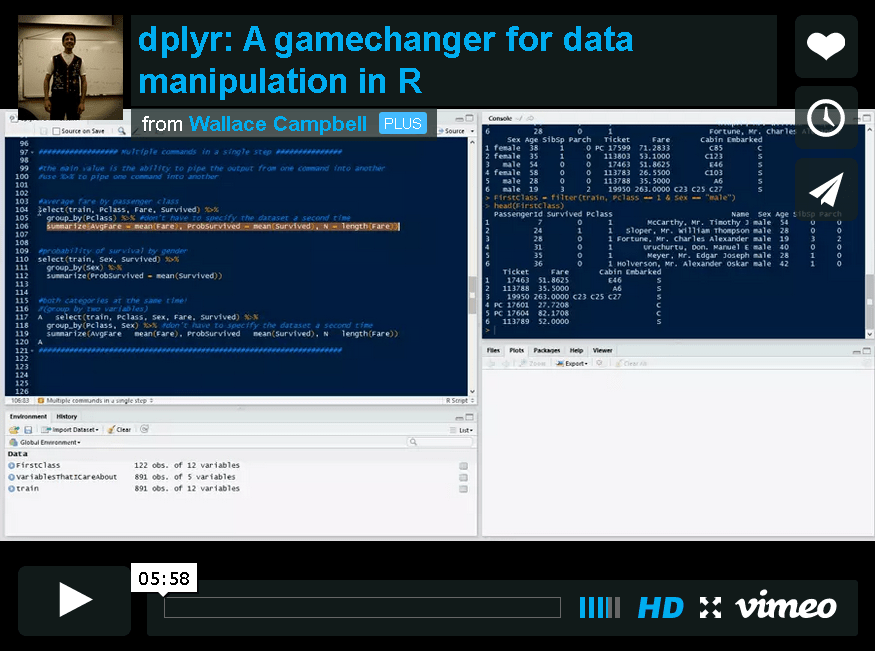# Blog Archives

## dplyr: A gamechanger for data manipulation in R

August 19, 2014
ByI demonstrate how to use dplyr for data manipulation in R (R code and data on GitHub ). I had heard of the package before and finally gave it a try after attending Hadley Wickham's presentation at useR! in LA a couple of months ago. dplyr will change y...

## GBMs are awesome: Part I

August 19, 2014
By

GBMs have become my favorite type of model over the last two years. In this tutorial, I demonstrate how to use a GBM for binary classification in R (predicting whether an event occurs or not). I also discuss basic model tuning and model inference with ...

## Analysis of experimental data with R

December 21, 2013
By

It's been a while since my last video, with good reason: I started a full time job as a senior statistician about 2 months ago! Not that I spend my entire day coding in R, but I wouldn't be nearly as useful if I didn't know how to use it. Anyway, whil...

## K-Fold Cross validation: Random Forest vs GBM

September 25, 2013
By

K-Fold Cross validation: Random Forest vs GBM from Wallace Campbell on Vimeo. In this video, I demonstrate how to use k-fold cross validation to obtain a reliable estimate of a model's out of sample predictive accuracy as well as compare two different types of models (a Random Forest and a GBM). I use data Kaggle's Amazon competition as...

## Multicore (parallel) processing in R

August 27, 2013
By

Multicore (parallel) processing in R from Wallace Campbell on Vimeo. If you're not programming in parallel, you're only using a fraction of your computer's power! I demonstrate how to run "for" loops in parallel using the mclapply function from the multicore library. The code can be scaled to any number of available cores.

## Survival analysis in R: Weibull and Cox proportional hazards models

August 26, 2013
By

I describe how to estimate the Weibull accelerated failure time model and the Cox proportional hazards model, test the assumptions, make predictions, and plot survival functions using each model. Survival analysis in R: Weibull and Cox proportional haz...

## Using a GBM for Classification in R

August 26, 2013
By

I discuss some advantages of Generalized Boosted Models over logistic regression and discriminant analysis and demonstrate how to use a GBM for binary classification (predicting whether an event occurs or not). Using a GBM for Classification in R from...

## Nonparametric (local polynomial) regression in R

August 26, 2013
By

Local polynomial regression models can be used as a more flexible alternative to linear regression. However, the nonparametric regression models are slightly more difficult to estimate and interpret than linear regression. This video explains almost ev...

## Estimation, prediction, and evaluation of logistic regression models

August 26, 2013
By

I provide an introduction to using logistic regression for prediction (binary classification) using the Titanic data competition from www.Kaggle.com as an example. I use models to predict in missing data, estimate a logistic regression model on a trai...

## R Video Blog! 2013-08-26 08:39:00

August 26, 2013
By

For more R resources, check out R-Bloggers! I seriously learn something every day from this site.